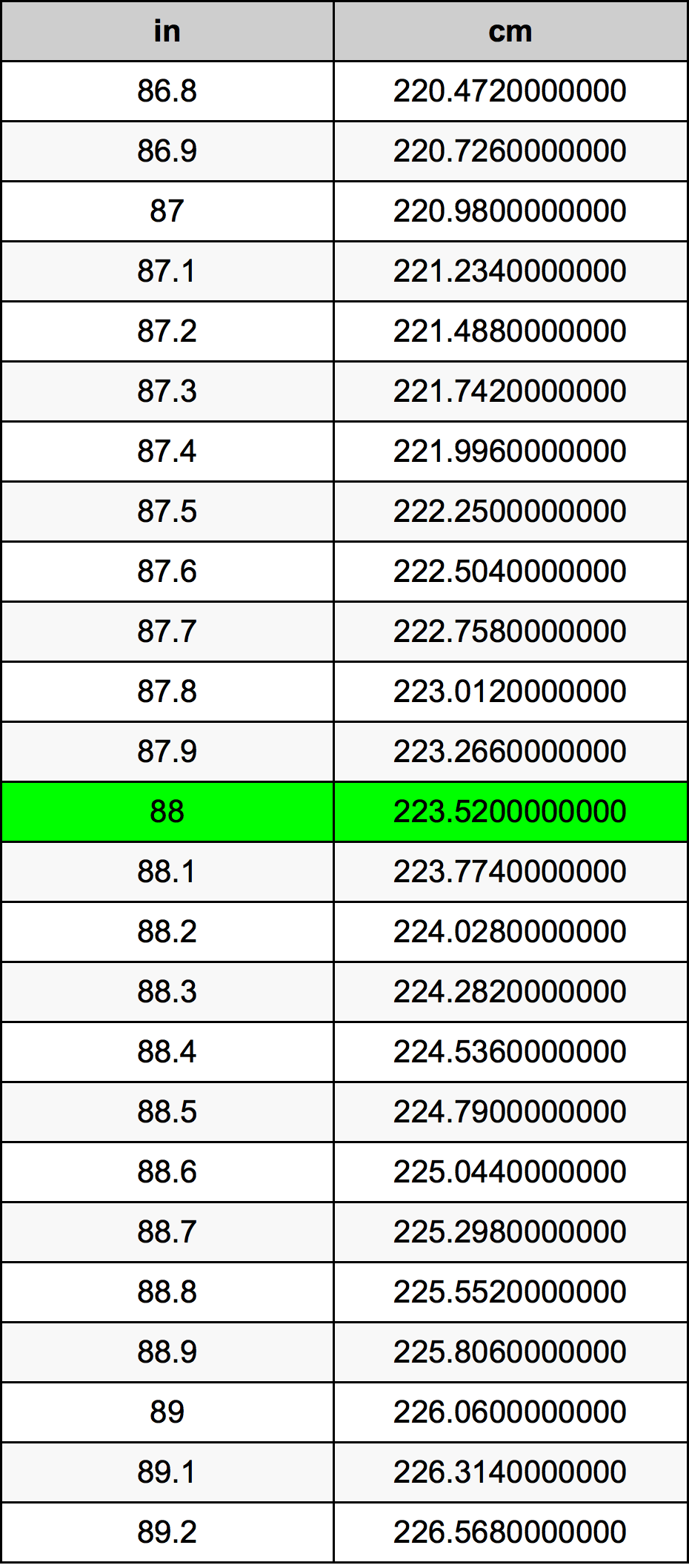Inches To Centimeters

# 88 in to cm88 Inches to Centimeters

in
=
cm

## How to convert 88 inches to centimeters?

 88 in * 2.54 cm = 223.52 cm 1 in
A common question is How many inch in 88 centimeter? And the answer is 34.6456692913 in in 88 cm. Likewise the question how many centimeter in 88 inch has the answer of 223.52 cm in 88 in.

## How much are 88 inches in centimeters?

88 inches equal 223.52 centimeters (88in = 223.52cm). Converting 88 in to cm is easy. Simply use our calculator above, or apply the formula to change the length 88 in to cm.

## Convert 88 in to common lengths

UnitUnit of length
Nanometer2235200000.0 nm
Micrometer2235200.0 µm
Millimeter2235.2 mm
Centimeter223.52 cm
Inch88.0 in
Foot7.3333333333 ft
Yard2.4444444444 yd
Meter2.2352 m
Kilometer0.0022352 km
Mile0.0013888889 mi
Nautical mile0.0012069114 nmi

## What is 88 inches in cm?

To convert 88 in to cm multiply the length in inches by 2.54. The 88 in in cm formula is [cm] = 88 * 2.54. Thus, for 88 inches in centimeter we get 223.52 cm.

## 88 Inch Conversion Table## Alternative spelling

88 Inches to cm, 88 Inches in cm, 88 Inch to Centimeters, 88 Inch in Centimeters, 88 in to cm, 88 in in cm, 88 in to Centimeter, 88 in in Centimeter, 88 Inches to Centimeter, 88 Inches in Centimeter, 88 Inches to Centimeters, 88 Inches in Centimeters, 88 Inch to cm, 88 Inch in cm